﻿ 可见区分光光度法测定水体色度的优化与应用 Improvement and Application of Spectrophotometry Method to Determinate Water Colority in Visible Spectrum

Journal of Advances in Physical Chemistry
Vol. 07  No. 04 ( 2018 ), Article ID: 27458 , 10 pages
10.12677/JAPC.2018.74021

Improvement and Application of Spectrophotometry Method to Determinate Water Colority in Visible Spectrum

Yuying Xie, Zuliang Chen*, Zhaolong Li, Yuehong Zhang, Huaming Wang, Meiling Shen

Zhejiang Institute of Application Energy and Nuclear Technology, Hangzhou ZhejiangReceived: Oct. 18th, 2018; accepted: Nov. 1st, 2018; published: Nov. 8th, 2018ABSTRACT

An improved universal method of determining the water colority of any kind is presented by numerical simulation between colority of Pt-Co standard solution and its chromatic tri-stimulus in visible region. It is recommended to simulate within 0 Hazen to 100 Hazens of Pt-Co standard solutions, by comparing simulations within different range of Pt-Co’s colority. By comparing CIE standard colorimetric observer matching functions with spectrum of Pt-Co standard solutions, it is found that relevance of C-Q simulation is more associated with C-Z than C-X or C-Y. At the meantime, simulations of C-X and C-Y during wave range of 560 - 780 nm show poor relevance which will reduce the accuracy of colority calculating. By removing this wave band while simulating, the accuracy of colority calculating will be improved effectively.

Keywords:Colorimetry, Colority, Tri-Stimulus Values, Spectrophotometry, Colorimetric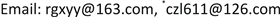1. 引言

2. 色度测定方法

2.1. 基于色度学的分光光度法

$Y=k{\sum }_{{\lambda }_{1}}^{{\lambda }_{2}}S\left(\lambda \right)\tau \left(\lambda \right)V\left(\lambda \right)\Delta \lambda$ (1)

$k=\frac{100}{{\sum }_{{\lambda }_{1}}^{{\lambda }_{2}}S\left(\lambda \right)V\left(\lambda \right)\Delta \lambda }$ (2)

${\lambda }_{1}$${\lambda }_{2}$ 为光谱扫描的起始波长和结束波长。

$\left\{\begin{array}{l}X=k{\sum }_{{\lambda }_{1}}^{{\lambda }_{2}}S\left(\lambda \right)\tau \left(\lambda \right)\overline{X}\left(\lambda \right)\Delta \lambda \\ Y=k{\sum }_{{\lambda }_{1}}^{{\lambda }_{2}}S\left(\lambda \right)\tau \left(\lambda \right)\overline{y}\left(\lambda \right)\Delta \lambda \\ Z=k{\sum }_{{\lambda }_{1}}^{{\lambda }_{2}}S\left(\lambda \right)\tau \left(\lambda \right)\overline{Z}\left(\lambda \right)\Delta \lambda \end{array}$ (3)

$Q=\sqrt{{\left({X}_{n}-X\right)}^{2}+{\left({Y}_{n}-Y\right)}^{2}+{\left({Z}_{n}-Z\right)}^{2}}$ (4)

$\left\{\begin{array}{l}{X}_{n}=94.81\\ {Y}_{n}=100.0\\ {Z}_{n}=107.33\end{array}$ (5)

2.2. 实验器材和实验方法

2.2.1. 材料与设备

2.2.2. 实验方法

1) 根据国家标准配制色度500度的标准储备液：将1.245 ± 0.0019克氯铂酸钾K2PtCl6和1.000 ± 0.0019克六水氯化钴(CoCl2⋅6H2O)溶于约500 mL超纯水中，加100 ± 1 mL盐酸(密度为1.18 g/ml)，并在1000 mL的容量瓶内用超纯水稀释至标线，避光静置12小时。

2) 采用1 cm的石英比色皿在分光光度计中测出500度标准储备液的吸光度(或透射率)，如果吸光度(或透射率)落入标准GB/T 9282.1-88  范围，则该500度原液符合标准，可使用。

3) 按国家标准配制标准溶液，将500度原液稀释成两组溶液。① 5、10、15、20、25、30、35、40、50、60、70、80、90；② 5、20、40、60、80、100、150、200、250、300、350、400、450、500度。

4) 为了提高精度，本文使用100 mm光程的比色皿测量以上标准溶液的吸光度，波长扫描间隔为5 nm。

3. 可见区分光光度法测色度

3.1. 标准储备液吸光度验证Table 1. Mensurated absorbance and allowed absorbance of 500 Hazens Pt-Co standard solution

3.2. 可见区分光光度法的色度范围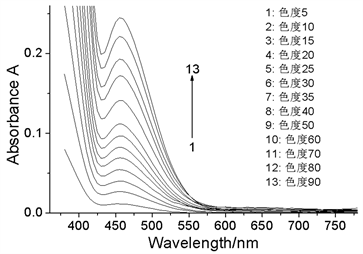(a) 色度范围为0~500度 (b) 色度范围为0~100度

Figure 1. Spectral curve of Pt-Co standard solution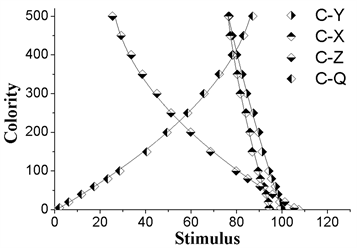(a)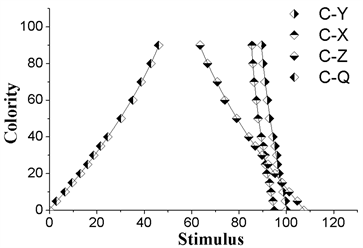(b)

Figure 2. Relationship between tri-stimulus values and colority fitting in different colority. (a) Colority range: 0 - 500 Hazens; (b) colority range: 0 - 100 Hazens

$\left\{\begin{array}{l}C=6480.6-120.7X+0.55{X}^{2}\text{\hspace{0.17em}}\text{\hspace{0.17em}}\text{\hspace{0.17em}}\text{\hspace{0.17em}}{R}^{2}=0.9966\\ C=3562.2-54.7Y+0.19{Y}^{2}\text{\hspace{0.17em}}\text{\hspace{0.17em}}\text{\hspace{0.17em}}\text{\hspace{0.17em}}\text{\hspace{0.17em}}\text{\hspace{0.17em}}\text{\hspace{0.17em}}{R}^{2}=0.9982\\ C=769.8-12.6Z+0.05{Z}^{2}\text{\hspace{0.17em}}\text{\hspace{0.17em}}\text{\hspace{0.17em}}\text{\hspace{0.17em}}\text{\hspace{0.17em}}\text{ }\text{\hspace{0.17em}}\text{ }\text{\hspace{0.17em}}\text{\hspace{0.17em}}{R}^{2}=0.9971\\ C=7.8+1.8Q+0.04{Q}^{2}\text{\hspace{0.17em}}\text{\hspace{0.17em}}\text{\hspace{0.17em}}\text{\hspace{0.17em}}\text{\hspace{0.17em}}\text{\hspace{0.17em}}\text{\hspace{0.17em}}\text{\hspace{0.17em}}\text{\hspace{0.17em}}\text{\hspace{0.17em}}\text{\hspace{0.17em}}\text{ }\text{ }\text{\hspace{0.17em}}\text{\hspace{0.17em}}{R}^{2}=0.9978\end{array}$ (6)

$\left\{\begin{array}{l}C=4570.9-91.9X+0.46{X}^{2}\text{\hspace{0.17em}}\text{\hspace{0.17em}}\text{\hspace{0.17em}}\text{\hspace{0.17em}}\text{\hspace{0.17em}}{R}^{2}=0.9900\\ C=3559.8-66.3Y+0.31{Y}^{2}\text{\hspace{0.17em}}\text{\hspace{0.17em}}\text{\hspace{0.17em}}\text{\hspace{0.17em}}\text{\hspace{0.17em}}\text{\hspace{0.17em}}\text{\hspace{0.17em}}{R}^{2}=0.9930\\ C=321.4-4.7Z+0.02{Z}^{2}\text{\hspace{0.17em}}\text{\hspace{0.17em}}\text{\hspace{0.17em}}\text{\hspace{0.17em}}\text{\hspace{0.17em}}\text{\hspace{0.17em}}\text{ }\text{\hspace{0.17em}}\text{\hspace{0.17em}}\text{\hspace{0.17em}}{R}^{2}=0.9990\\ C=1.3+1.2Q+0.02{Q}^{2}\text{\hspace{0.17em}}\text{\hspace{0.17em}}\text{\hspace{0.17em}}\text{\hspace{0.17em}}\text{\hspace{0.17em}}\text{\hspace{0.17em}}\text{\hspace{0.17em}}\text{\hspace{0.17em}}\text{\hspace{0.17em}}\text{\hspace{0.17em}}\text{\hspace{0.17em}}\text{\hspace{0.17em}}\text{ }\text{\hspace{0.17em}}{R}^{2}=0.9985\end{array}$ (7)

(6)式对应色度范围为0~500，(7)式对应色度范围为0~500，其中R2为拟合相关度。观察上述拟合关系可以发现，C-X相关度最低，C-Y好之，而C-Z是三者中相关度最好的。对比C-Q与C-X、C-Y、C-Z关系可以发现，C-Q关系的相关度整体上要好于X、Y单独拟合的相关度，与C-Z接近，可见采用三者方差的形式可以有效的降低误差，提高拟合的准确度。而C-Q的拟合准度受C-Z影响要更为明显，我们知道Z值对色度的响应比X、Y更为明显，在式(3)中就体现为 ${\left({Z}_{n}-Z\right)}^{2}$ 的数值比另两项更大，因此整体上表现出C-Q关系与C-Z关系关联程度更高。观察上述关系还可以发现0~100色度范围下C-Q的拟合精度要好于0~500色度范围下的拟合。将两组标样的三刺激值回代入(6)、(7)式可以计算出这一系列数值关系下标样的色度值，我们列出了普适性关系C-Q下两组标样的色度值，如表2所示。Table 2. Numeric value and standard colority of Pt-Co standard solution fitting in different colority

3.3. 可见区分光光度法的波长范围

$\left\{\begin{array}{l}X\left(\lambda \right)=k\ast S\left(\lambda \right)\ast \overline{X}\left(\lambda \right)\ast \Delta \lambda \\ Y\left(\lambda \right)=k\ast S\left(\lambda \right)\ast \overline{y}\left(\lambda \right)\ast \Delta \lambda \\ Z\left(\lambda \right)=k\ast S\left(\lambda \right)\ast \overline{Z}\left(\lambda \right)\ast \Delta \lambda \end{array}$ (8)Figure 3. Comparison of function X(λ), Y(λ), Z(λ) and absorbance curve A(λ)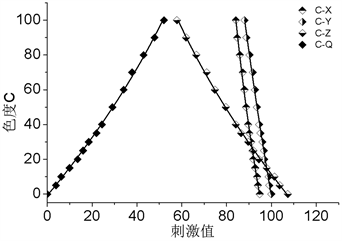(a)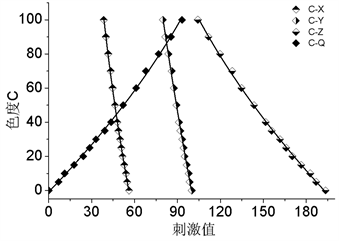(b)

Figure 4. Relationship between tri-stimulus values and colority fitting in different wavelength. (a) Wavelength range: 380 - 780 nm; (b) wavelength range: 380 - 560 nm

$\left\{\begin{array}{l}C=0.22{X}^{2}-49.1X+2658.8\text{\hspace{0.17em}}\text{\hspace{0.17em}}\text{\hspace{0.17em}}\text{\hspace{0.17em}}\text{\hspace{0.17em}}{R}^{2}=0.9962\\ C=0.085{Y}^{2}-24.4Y+1593.2\text{\hspace{0.17em}}\text{\hspace{0.17em}}\text{\hspace{0.17em}}\text{\hspace{0.17em}}\text{\hspace{0.17em}}\text{ }{R}^{2}=0.9966\\ C=0.011{Z}^{2}-3.8Z+285.3\text{\hspace{0.17em}}\text{\hspace{0.17em}}\text{\hspace{0.17em}}\text{\hspace{0.17em}}\text{\hspace{0.17em}}\text{\hspace{0.17em}}\text{\hspace{0.17em}}\text{\hspace{0.17em}}\text{ }\text{ }{R}^{2}=0.9996\\ C=0.0097{Q}^{2}+1.43Q\text{\hspace{0.17em}}\text{\hspace{0.17em}}\text{\hspace{0.17em}}\text{\hspace{0.17em}}\text{\hspace{0.17em}}\text{\hspace{0.17em}}\text{\hspace{0.17em}}\text{\hspace{0.17em}}\text{\hspace{0.17em}}\text{\hspace{0.17em}}\text{\hspace{0.17em}}\text{\hspace{0.17em}}\text{\hspace{0.17em}}\text{\hspace{0.17em}}\text{ }\text{\hspace{0.17em}}\text{ }{R}^{2}=0.9995\end{array}$ (9)

$\left\{\begin{array}{l}C=0.078{X}^{2}-13.1X+490.1\text{\hspace{0.17em}}\text{\hspace{0.17em}}\text{\hspace{0.17em}}\text{\hspace{0.17em}}\text{\hspace{0.17em}}\text{\hspace{0.17em}}{R}^{2}=0.9994\\ C=0.028{Y}^{2}-10.0Y+723.2\text{\hspace{0.17em}}\text{\hspace{0.17em}}\text{\hspace{0.17em}}\text{\hspace{0.17em}}\text{\hspace{0.17em}}\text{\hspace{0.17em}}\text{\hspace{0.17em}}{R}^{2}=0.9987\\ C=0.003{Z}^{2}-2.1Z+285.3\text{\hspace{0.17em}}\text{\hspace{0.17em}}\text{\hspace{0.17em}}\text{\hspace{0.17em}}\text{\hspace{0.17em}}\text{\hspace{0.17em}}\text{\hspace{0.17em}}\text{\hspace{0.17em}}\text{ }{R}^{2}=0.9996\\ C=0.003{Q}^{2}+0.80Q\text{\hspace{0.17em}}\text{\hspace{0.17em}}\text{\hspace{0.17em}}\text{\hspace{0.17em}}\text{\hspace{0.17em}}\text{\hspace{0.17em}}\text{\hspace{0.17em}}\text{\hspace{0.17em}}\text{\hspace{0.17em}}\text{\hspace{0.17em}}\text{\hspace{0.17em}}\text{\hspace{0.17em}}\text{\hspace{0.17em}}\text{\hspace{0.17em}}\text{\hspace{0.17em}}\text{\hspace{0.17em}}\text{\hspace{0.17em}}\text{ }{R}^{2}=0.9996\end{array}$ (10)

3.4. 可见区分光光度法测定实际水样色度验证Table 3. Numeric value Q and standard colority of Pt-Co standard solution fitting in different wavelength: 380 – 780 nm, 380 - 560 nmTable 4. Colority of samples mensurated by three methods: Eye-measurement, Spectrophotometry (original), Spectrophotometry (improved)

4. 结论

1) 利用色度学知识，将铂–钴标准溶液在可见光区的吸光度值转化为三刺激值，建立色度与三刺激值方差之间的数值关系，这一方法可以用于任意水体色度的测定。

2) 针对这一方法所采用的铂–钴标准溶液的色度范围进行了实验对比，发现色度范围的缩小，将显著提高色度测定的准度，为了便于水样测试，同时保证准度，我们将色度范围设置在0~100度。

3) 对比XYZ空间颜色匹配函数与铂–钴标准溶液吸光度曲线和 $\tau \left(\lambda \right)$ 的重叠情况，得知C-Q数值关系的相关度主要由C-Z关系决定。而在实际测量水体的色度时发现，色度测定的准度由XYZ空间所有参量共同决定，而铂钴溶液的特性使得C-X、C-Y关系相关度偏低，结合理论分析发现去除560~780 nm这一长波波段的干扰，将有效提高C-X、C-Y相关度，从而提高色度测定的精度。经对比实验证实，这一优化将使分光光度法测定色度的精度有明显提升。

Improvement and Application of Spectrophotometry Method to Determinate Water Colority in Visible Spectrum[J]. 物理化学进展, 2018, 07(04): 174-183. https://doi.org/10.12677/JAPC.2018.74021

1. 1. GB 11903-1989水质色度的测定[S].

2. 2. 郜洪文. 分光光度法测定工业废水色度研究[J]. 环境工程, 1993, 11(5): 44-47.

3. 3. 刘春, 郭春娟. 用分光光度计测定地面水色度[J]. 环境科技, 1994, 14(1): 75-76.

4. 4. 马登军. 分光光度法快速测定天然水色度[J]. 中国环境监测, 1993, 9(4): 15-16.

5. 5. 王永琴, 苏筱军. 分光光度法测定水中色度探讨[J]. 预防医学, 1997, 3(2): 146.

6. 6. 于夕娟. 水中色度的分光光度测定法[J]. 职业与健康, 2008, 24(15): 1515-1516.

7. 7. 曾凡亮, 罗仙桃. 分光光度法测定水样的色度[J]. 工业水处理, 2006, 26(9): 69-73.

8. 8. 毛善巧, 杨锋. 分光光度法测定水力空化对绿原酸的影响[J]. 中国食品添加剂, 2017(6): 184-189.

9. 9. 陈洪雷, 杨桂花. 比值–导数光谱法测定APMP制浆废水的色度[J]. 林产化学与工业, 2014, 34(5): 35-40.

10. 10. 陈强, 罗亮. 分光光度法测定水体色度的一种新方法[J]. 甘肃环境研究与监测, 2003, 16(4): 342-343.

11. 11. 余潘, 沈为民, 黄杰, 等. 可见区分光光度法测量水体色度[J]. 光学技术, 2011, 37(5): 551-555.

12. 12. 黄杰, 沈为民, 楼俊, 等. 基于光谱及色差计算测定水质的色度[J]. 光谱实验室, 2012, 29(6): 3399-3403.

13. 13. 沈为民, 余潘, 黄杰, 等. 水质色度的色差测量方法[J]. 计量学报, 2013, 34(4): 320-324.

14. 14. GB/T 3978-2008标准照明体及照明观察条件[S].

15. 15. 汤顺青. 波长范围对色度学计算精度的影响[J]. 光学技术, 1998(2): 58-60.

16. 16. GB/T 3977-2008 颜色的表示方法[S].

17. 17. GB 9282.1-88透明液体以铂一钻等级评定颜色[S].

NOTES

*通讯作者。